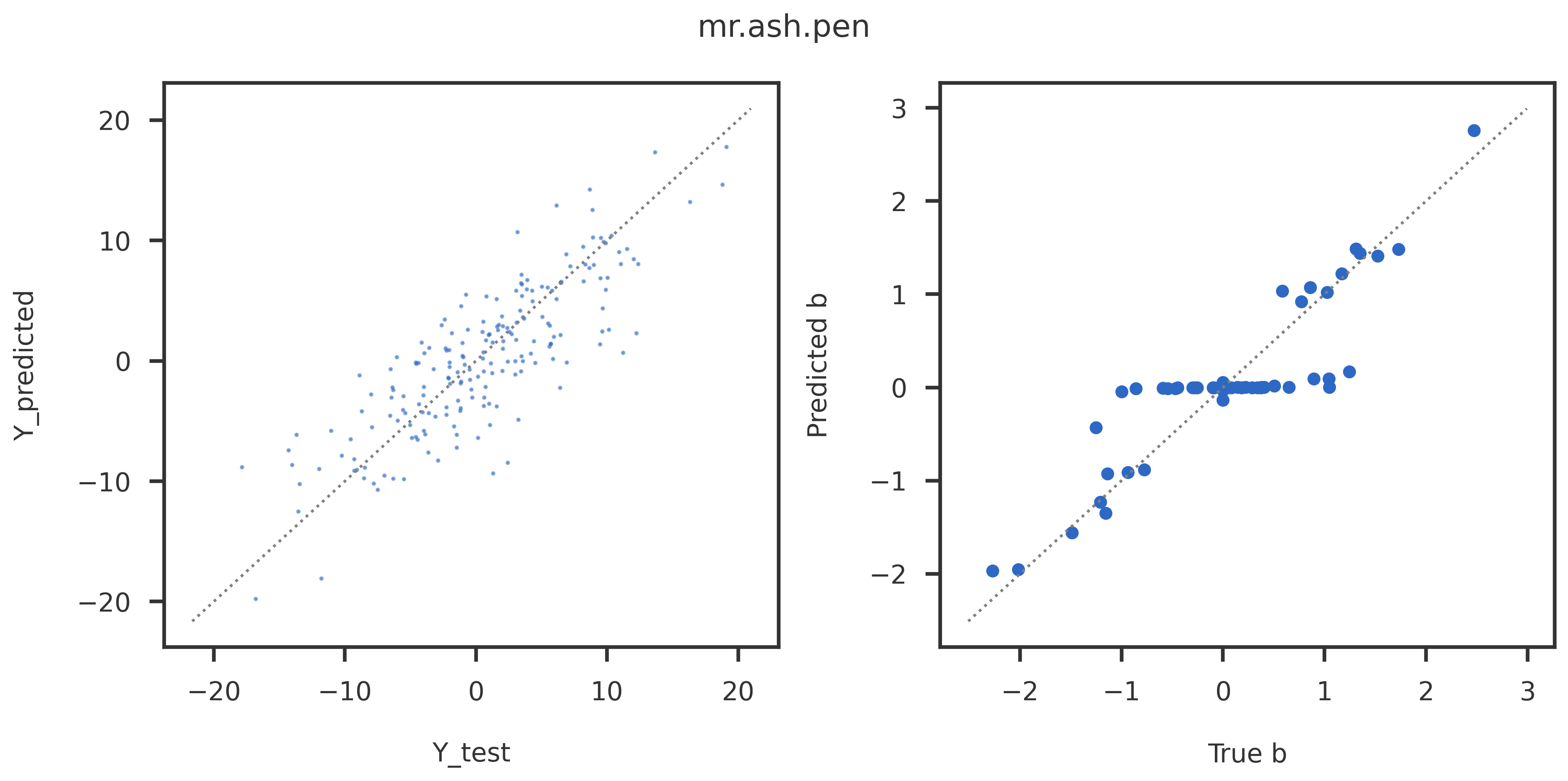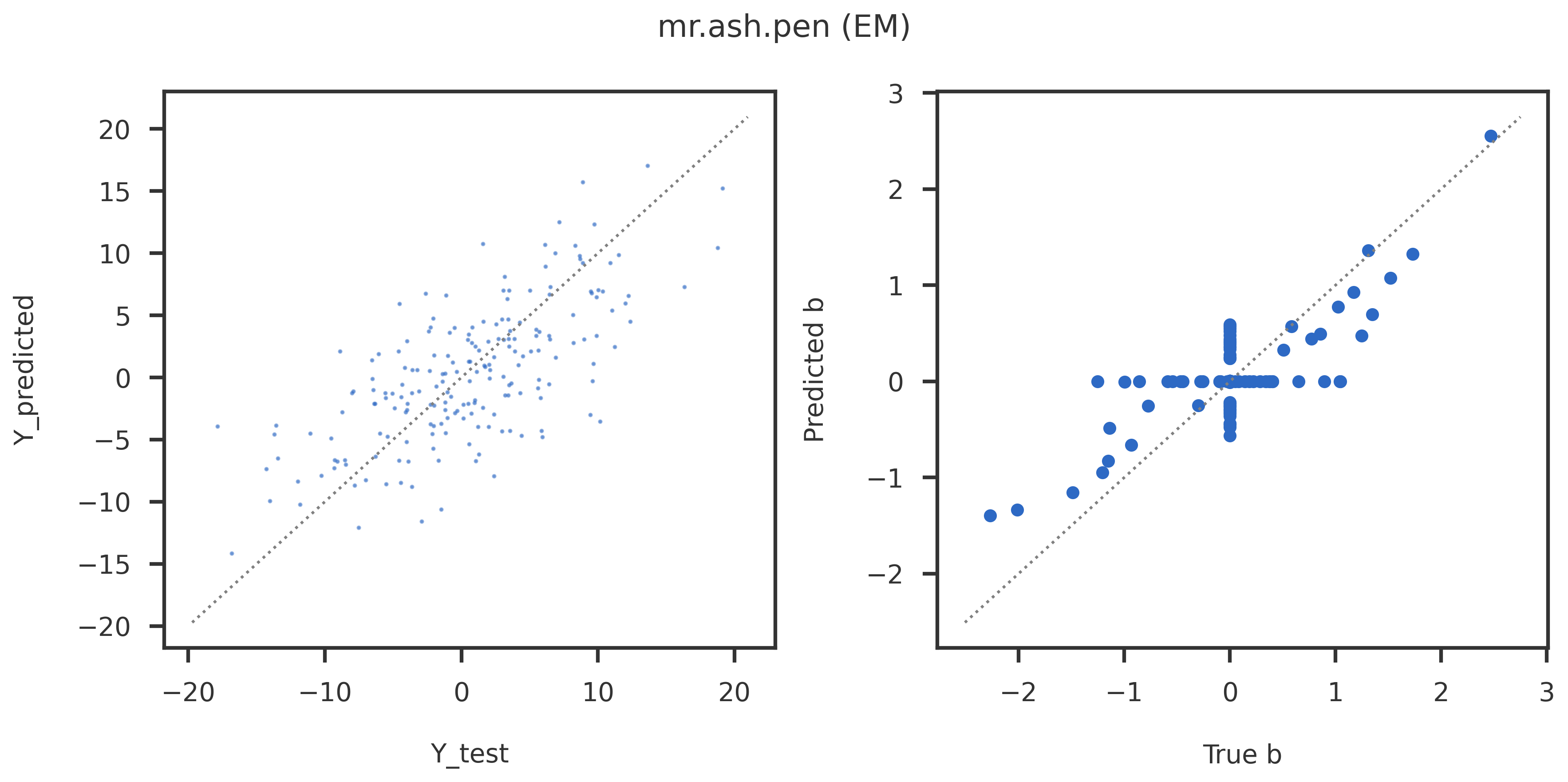In this demo, I illustrate the convergence of three different algorithms for using Mr.ASH in sparse multiple linear regression and trendfiltering. Given response variables $\mathbf{y}$ for $N$ samples and explanatory variables $\mathbf{X}$ for $P$ variables (generally $N \lt P$). We will perform a sparse multiple regression using the adaptive shrinkage prior (Mr.ASH),

$\mathbf{y} = \mathbf{X}\mathbf{b} + \mathbf{e}$,

$\mathbf{e} \sim \mathcal{N}\left(\mathbf{0} \mid \sigma^2 I_n \right)$,

$\mathbf{b} \sim p\left(\mathbf{b} \mid \boldsymbol{\theta}_1\right)$.

$p\left(b_i \mid \boldsymbol{\theta}_1\right) = \sum_{k=1}^{K} w_k \mathcal{N}\left(b_i \mid \mu_k, \sigma_k^2\right)$ with constraints $w_k \ge 0$, $\sum_{k=1}^{K} w_k = 1$

We assume $\sigma_k$ is known and will estimate ($\mathbf{b}, \mathbf{w}, \sigma^2)$ from the data.

Here, I will compare four methods:

• mr.ash.alpha. Co-ordinate ascent algorithm for maximizing ELBO (as implemented in mr.ash.alpha; Github)
• mr.ash.pen. Penalized linear regression using gradient descent (L-BFGS-B) algorithm (as implemented in mr.ash.pen; Github).
• mr.ash.pen (EM). Hybrid algorithm which iterates between (i) estimating $\mathbf{b}$ by minimizing PLR objective (approzimate E-Step), and (ii) estimating $\mathbf{w}$ and $\sigma^2$ by maximizing ELBO (approximate M-step).
• mr.ash.alpha (init). Same as mr.ash.alpha, but initialized with the results from mr.ash.pen.

I will illustrate four examples, where mr.ash.alpha is known to perform poorly.

Note: I will use the ELBO at each iteration for comparing the methods, although it is to be noted that the objective function for mr.ash.pen and the objective function for the E-step of mr.ash.pen (EM) are different from the ELBO. Hence, the ELBO will not be monotonically increasing for these two methods. Also, the varobj obtained from mr.ash.alpha is an approximation to the true ELBO and matches with the true ELBO close to the optimum.

import numpy as np
import pandas as pd

from mrashpen.inference.penalized_regression import PenalizedRegression as PLR
from mrashpen.inference.mrash_wrapR          import MrASHR
from mrashpen.models.plr_ash                 import PenalizedMrASH
from mrashpen.models.normal_means_ash_scaled import NormalMeansASHScaled
from mrashpen.inference.ebfit                import ebfit
from mrashpen.inference                      import lbfgsfit
from mrashpen.utils                          import R_lasso
from mrashpen.models                         import mixture_gaussian as mix_gauss

import sys
sys.path.append('/home/saikat/Documents/work/sparse-regression/simulation/eb-linreg-dsc/dsc/functions')
import simulate

import matplotlib.pyplot as plt
from pymir import mpl_stylesheet
from pymir import mpl_utils
mpl_stylesheet.banskt_presentation(splinecolor = 'black')

def center_and_scale(Z):
dim = Z.ndim
if dim == 1:
Znew = Z / np.std(Z)
Znew = Znew - np.mean(Znew)
elif dim == 2:
Znew = Z / np.std(Z, axis = 0)
Znew = Znew - np.mean(Znew, axis = 0).reshape(1, -1)
return Znew

def initialize_ash_prior(k, scale = 2, sparsity = None):
w = np.zeros(k)
w = 1 / k if sparsity is None else sparsity
w[1:(k-1)] = np.repeat((1 - w)/(k-1), (k - 2))
w[k-1] = 1 - np.sum(w)
sk2 = np.square((np.power(scale, np.arange(k) / k) - 1))
prior_grid = np.sqrt(sk2)
return w, prior_grid

def plot_linear_mrashpen(X, y, Xtest, ytest, btrue, strue, bhat,
intercept = 0, title = None):
ypred = np.dot(Xtest, bhat) + intercept
fig = plt.figure(figsize = (12, 6))
ax1.scatter(ytest, ypred, s = 2, alpha = 0.5)
mpl_utils.plot_diag(ax1)
ax2.scatter(btrue, bhat)
mpl_utils.plot_diag(ax2)

ax1.set_xlabel("Y_test")
ax1.set_ylabel("Y_predicted")
ax2.set_xlabel("True b")
ax2.set_ylabel("Predicted b")
if title is not None:
fig.suptitle(title)
plt.tight_layout()
plt.show()

def plot_convergence(objs, methods, nwarm, eps = 1e-8):
fig = plt.figure(figsize = (12, 6))

objmin  = np.min([np.min(x) for x in objs])

for obj, method, iteq in zip(objs, methods, nwarm):
m_obj = obj[iteq:] - objmin
m_obj = m_obj[m_obj > 0]
ax1.plot(range(iteq, len(m_obj) + iteq), np.log10(m_obj), label = method)
ax1.legend()

ax1.set_xlabel("Number of Iterations")
ax1.set_ylabel("log( max(ELBO) - ELBO )")

plt.show()
return

def plot_trendfilter_mrashpen(X, y, beta, ytest, bhat,
intercept = 0, title = None):
n = y.shape
p = X.shape

ypred = np.dot(X, bhat) + intercept
fig = plt.figure(figsize = (12, 6))
ax1.scatter(np.arange(n), ytest, edgecolor = 'black', facecolor='white')
ax1.plot(np.arange(n), ypred)
ax1.set_xlabel("Sample index")
ax1.set_ylabel("Y")

ax2.scatter(np.arange(p), beta, edgecolor = 'black', facecolor = 'white')
ax2.scatter(np.arange(p), bhat, s = 40, color = 'firebrick')
ax2.set_xlabel("Sample index")
ax2.set_ylabel("b")

if title is not None:
fig.suptitle(title)

plt.tight_layout()
plt.show()

def linreg_summary_df(sigma2, objs, methods):
data     = [[strue * strue,  '-', '-']]
rownames = ['True']
for obj, method in zip(objs, methods):
data.append([obj.residual_var, obj.elbo_path[-1], obj.niter])
rownames.append(method)
colnames = ['sigma2', 'ELBO', 'niter']
df = pd.DataFrame.from_records(data, columns = colnames, index = rownames)
return df


## 1. Dense, high-dimensional setting

In high-dimensional data ($N = 200$ and $P = 2000$), Mr.ASH is known perform worse than LASSO in a dense setting with large number of non-causal variables and high PVE (1, 2). Here, I used $P_{\textrm{causal}} = 500$ and PVE $= 0.95$. Here, mr.ash.pen and mr.ash.pen (EM) converges to a better solution, the first one with a significantly higher ELBO and the second one with a slightly higher ELBO (!).

Note: I found that mr.ash.pen (EM) converges to the correct solution for a wider range of $P_{\textrm{causal}}$. (To do: Run simulation with DSC to explore the full range)

n = 200
p = 2000
p_causal = 50
pve = 0.95
k = 20

X, y, Xtest, ytest, btrue, strue = simulate.equicorr_predictors(n, p, p_causal, pve, rho = 0.0, seed = 100)
X      = center_and_scale(X)
Xtest  = center_and_scale(Xtest)
wk, sk = initialize_ash_prior(k, scale = 2)


Here, I run the three methods.

'''
Lasso initialization
'''
lasso_a0, lasso_b, _ = R_lasso.fit(X, y)
s2init = np.var(y - np.dot(X, lasso_b) - lasso_a0)
winit  = mix_gauss.emfit(lasso_b, sk)
print ("Lasso initialization")

'''
mr.ash.pen
'''
plr_lbfgs = PLR(method = 'L-BFGS-B', is_prior_scaled = True,
debug = False, display_progress = False, calculate_elbo = True,
maxiter = 2000, tol = 1e-8)
plr_lbfgs.fit(X, y, sk, binit = lasso_b, winit = winit, s2init = s2init)
# plr_lbfgs.fit(X, y, sk, binit = None, winit = wk, s2init = s2init)
# plr_lbfgs = lbfgsfit(X, y, sk, wk, binit = lasso_b, s2init = s2init, calculate_elbo = True)

'''
mr.ash.pen (EM)
'''
# plr_eb = ebfit(X, y, sk, wk, binit = None, s2init = 1, maxiter = 200, qb_maxiter = 100)
plr_eb = ebfit(X, y, sk, binit = lasso_b, winit = winit, s2init = s2init,
maxiter = 200, qb_maxiter = 50, calculate_elbo = True)

'''
mr.ash.alpha
'''
mrash_r = MrASHR(option = "r2py", debug = False)
mrash_r.fit(X, y, sk, binit = lasso_b, winit = winit, s2init = s2init)

'''
mr.ash.alpha (init)
'''
mrash_r_init = MrASHR(option = "r2py", debug = False)
mrash_r_init.fit(X, y, sk, binit = plr_lbfgs.coef, winit = plr_lbfgs.prior, s2init = plr_lbfgs.residual_var)

R[write to console]: Loading required package: Matrix


Lasso initialization
mr.ash.pen terminated at iteration 424.
mr.ash.pen (EM) terminated at iteration 200.
Mr.ASH terminated at iteration 287.
Mr.ASH terminated at iteration 13.


On the left panel I compare the predicted $\mathbf{y}_{\mathrm{test}}$ by the different methods (y-axis) with the true $\mathbf{y}_{\mathrm{test}}$ (x-axis). On the right panel, I compare the predicted coefficients (y-axis) with their true values (x-axis). The plot at the bottom shows the convergence of the different methods against the number of iteration.

plot_linear_mrashpen(X, y, Xtest, ytest, btrue, strue,
lasso_b, intercept = lasso_a0, title = 'Lasso')
plot_linear_mrashpen(X, y, Xtest, ytest, btrue, strue,
mrash_r.coef, intercept = mrash_r.intercept, title = 'mr.ash.alpha')
plot_linear_mrashpen(X, y, Xtest, ytest, btrue, strue,
plr_lbfgs.coef, intercept = plr_lbfgs.intercept, title = 'mr.ash.pen')
plot_linear_mrashpen(X, y, Xtest, ytest, btrue, strue,
plr_eb.coef, intercept = plr_eb.intercept, title = 'mr.ash.pen (EM)')
plot_linear_mrashpen(X, y, Xtest, ytest, btrue, strue,
mrash_r_init.coef, intercept = mrash_r_init.intercept, title = 'mr.ash.alpha (init)')

kinit   = [0, 0, 0]
objs    = [mrash_r.obj_path, plr_lbfgs.elbo_path, plr_eb.elbo_path]
methods = ["mr.ash.alpha", "mr.ash.pen", "mr.ash.pen (EM)"]
plot_convergence(objs, methods, kinit)